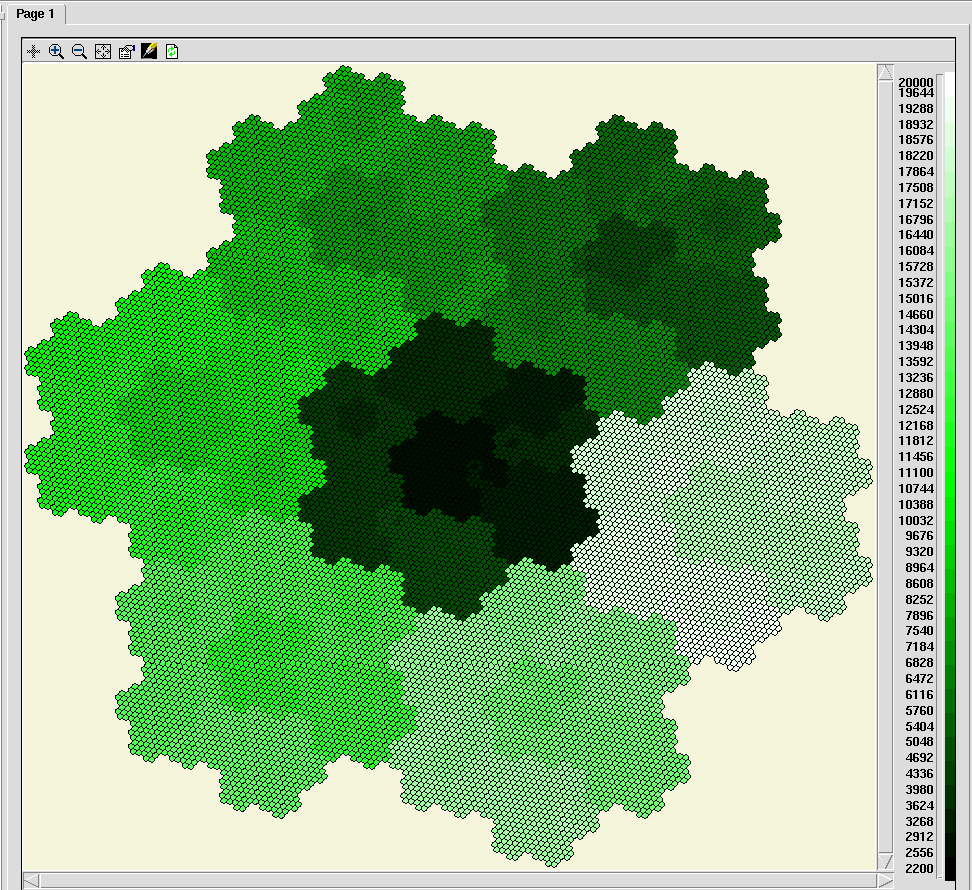# Exclusive Role

## A hierarchy of hexagonal tilings

ModelId:
hex_levels
SimileVersion:
4.0

This model has been included to illustrate a number of techniques used to create complex structures in which model components can interact. It allows an area to be represented as a series of hexagonal tilings, with increasing levels of detail. Each hexagon in one level corresponds to a group of 7 hexagons in the next level down. The arrangement is based on that used in the HOOFS model (S. P. Oom et al, 2004) It would be straightforward to implement this layout with a series of nested submodels, each representing one layer of the tiling.

Equations:

Equations in top level Variable magic magic = sqrt(3) Variable offa_even offa_even = [ 0.5, -0.5,-1, -0.5, 0.5,1] Variable offa_odd offa_odd = cos(theta)*[offa_even]-sin(theta)*[offb_even] Variable offb_even offb_even = [ 0.866, 0.866,0, -0.866, -0.866,0] Variable offb_odd offb_odd = sin(theta)*[offa_even]+cos(theta)*[offb_even] Variable theta theta = atan(3*sqrt(3)) Equations in hexagon Variable centre x centre x = if posn_in_parent==0 then hi_cx_lower else hi_cx_lower+magic*element(element([[offb]],my_level),int(posn_in_parent)) Where: my_level=my level posn_in_parent=posn in parent [[offb]]= ../level info/offb {hi_cx_higher}= ../hierarchy/hi_cx (to hexagon in higher) hi_cx_lower= ../hierarchy/hi_cx (to hexagon in lower) magic= ../magic Variable centre y centre y = if posn_in_parent==0 then hi_cy_lower else hi_cy_lower-magic*element(element([[offa]],my_level),int(posn_in_parent)) Where: my_level=my level posn_in_parent=posn in parent [[offa]]= ../level info/offa {hi_cy_higher}= ../hierarchy/hi_cy (to hexagon in higher) hi_cy_lower= ../hierarchy/hi_cy (to hexagon in lower) magic= ../magic Variable my level my level = hi_level_lower+1 Where: {hi_level_higher}= ../hierarchy/hi_level (to hexagon in higher) hi_level_lower= ../hierarchy/hi_level (to hexagon in lower) Variable my parent my parent = if index(1)==1 then 1 else int((index(1)+5)/7) Variable posn in parent posn in parent = if index(1)==1 then 0 else int(fmod(index(1)-2,7)) Equations in hierarchy Condition cond1 cond1 = index(1) is my_parent_lower Where: my_parent_lower= ../hexagon/my parent (from hexagon in lower) my_parent_higher= ../hexagon/my parent (from hexagon in higher) Variable dummy dummy = index(2)==1 Variable hi_cx hi_cx = if dummy then 0 else centre_x_higher Where: centre_x_lower= ../hexagon/centre x (from hexagon in lower) centre_x_higher= ../hexagon/centre x (from hexagon in higher) Variable hi_cy hi_cy = if dummy then 0 else centre_y_higher Where: centre_y_lower= ../hexagon/centre y (from hexagon in lower) centre_y_higher= ../hexagon/centre y (from hexagon in higher) Variable hi_level hi_level = if dummy then 0 else my_level_higher Where: my_level_lower= ../hexagon/my level (from hexagon in lower) my_level_higher= ../hexagon/my level (from hexagon in higher) Equations in level info Variable even level even level = fmod(index(1),2)== 1.0 Variable offa offa = side*(if even_level then [offa_even] else [offa_odd]) Where: even_level=even level [offa_even]= ../offa_even [offa_odd]= ../offa_odd Variable offb offb = side*(if even_level then [offb_even] else [offb_odd]) Where: even_level=even level [offb_even]= ../offb_even [offb_odd]= ../offb_odd Variable side side = 50/7^((index(1)-1)/2) Equations in for display Condition cond1 cond1 = my_level==6 Where: my_level= ../my level Variable colour colour = index(1) Variable xpts xpts = centre_x+element([[offa]],my_level) Where: centre_x= ../centre x [[offa]]= ../../level info/offa my_level= ../my level Variable ypts ypts = centre_y+element([[offb]],my_level) Where: centre_y= ../centre y [[offb]]= ../../level info/offb my_level= ../my level

Results:

Here is the diagram of the hexagons at the lowest level:References:

S.P. Oom, J.A. Beecham, C.J. Legg and A.J. Hester (2004) Foraging in a complex environment: from foraging strategies to emergent spatial properties     http://www.sciencedirect.com/science/article/pii/S1476945X04000522